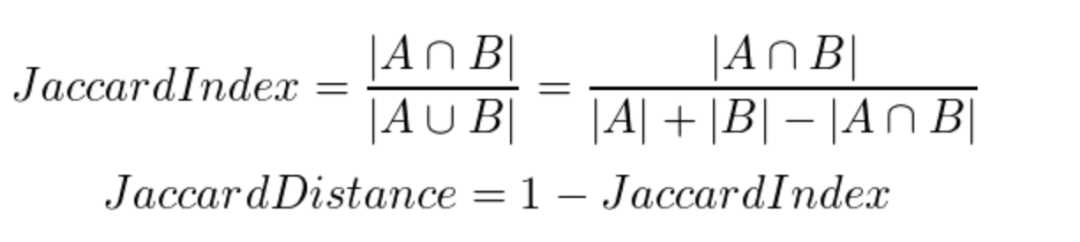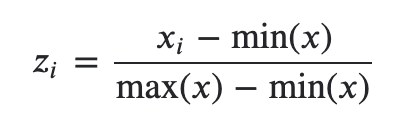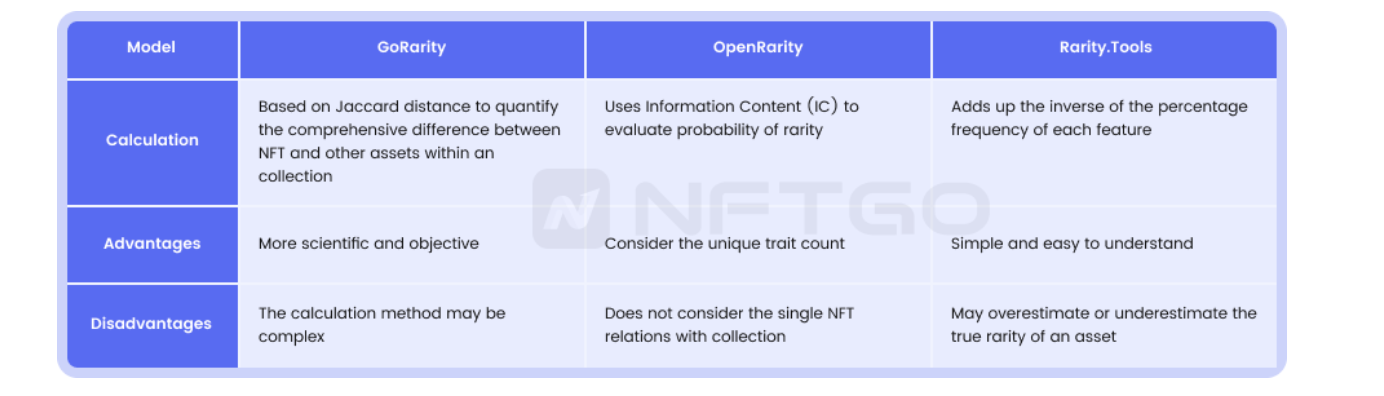# GoRarity

An open-source rarity model by NFTGo

### Introducing GoRarity### Problems and Solution

Non-Fungible Tokens (NFTs) are unique digital assets that differ from each other, and their rarity affects their value in the market. NFT rarity refers to how common a specific NFT is within a collection. Typically, the rarer the NFT, the higher its price. However, determining rarity and evaluating NFT value by rarity can be challenging due to the subjective nature of traditional rating scales. To address this issue, models like GoRarity emerged, which use mathematical models to calculate rarity levels based on categorized characteristics.

Profile Picture (PFP) projects dominate the NFT market and form a collection of different NFTs with each NFT being a unit of the whole. GoRarity's approach to rarity calculation emphasizes data aspects of an NFT and is more objective and transparent, and considers the rarity of each NFT in the context of the whole collection, which is important for PFP projects.

### GoRarity Model

GoRarity is an open-source rarity calculation model based on numerical models, designed to provide a more objective and accurate rarity ranking to users. It uses algorithms like Jaccard distance and normalization to reflect the relevance of an individual NFT to its original collection. The Jaccard distance algorithm is used to quantify the similarity between two sets of data. GoRarity uses this algorithm to evaluate the similarity between an NFT and its original collection. Normalization is used to adjust the rarity score based on the size of the original collection.

#### What is Jaccard distance?

Jaccard distance is a statistical method used to measure dissimilarity between sample sets ranging from 0 to 1. Its mathematical formula is as below.Jaccard distance is a common data science measure used to calculate the dissimilarity between objects. Its logic looks similar to that of a Venn diagram, measuring the size of the intersection between sample sets.

#### The calculation of GoRarity

NFTGo calculates the similarity of NFT traits based on Jaccard distance. As an example, let's calculate the rarity score of BAYC#1154:

• Calculate the Jaccard distance between #1154 and the other 9999 NFTs in the collection.
• Calculate the average of the Jaccard distances, which gives the initial data for the rarity score.
• Process the data from the previous step using the normalization method. The mathematical formula used is:• Multiply the z-score by 100 to get the final rarity score for BAYC#1154. The score is then ranked in a set (rarity scores range from 0 to 100) to get the final rarity ranking for that NFT.

### Why use GoRarity?

#### A more accurate reflection of true rarity

Many models for rarity calculation are available on the market. For example, OpenRarity chooses to use Information Content (IC) as a way to assess the probability of expression of rarity, while rarity.tools chooses to summarize the inversed percentage of the frequency in which each trait appears.

Experimental comparisons show that the rarity scores of other tools may overestimate or underestimate the rarity of an NFT, while GoRarity gives more reasonable results in these cases.

The following table shows the rarity evaluation models of GoRarity, OpenRarity, rarity.tools and NFTEXP, when calculating the rarity ranking of NFTs of the Bored Ape Yacht Club (BAYC) collection.

The fact that people have the same offer price for several NFTs in one collection often means that these NFTs are ranked close to each other in terms of rarity. We found that among the 15 BAYCs that share the same highest offer price, the ranking similarities of GoRarity and OpenRarity are considerably better than those of rarity.tools and NFTExp.io. Also in the comparison of some specific extreme values, GoRarity performs better.

Also, in the comparison of some specific extreme values, GoRarity performs better. GoRarity's rarity ranking is more advanced than OpenRarity's for #8027/#6583/#466, which have recently sold at the top price ranking.

To illustrate better, we will take rarity.tools as an example.

Taking BAYC #947 as an example, NFTGo ranks it as #9994, which is almost the least rare of all apes, with a rarity of 406. However, rarity.tools ranks it at #998, with a rarity score of 152.12. This is because rarity.tools simply summarizes each trait and the number of traits (yet it actually has no additional special traits). As shown in the figure below, this monkey has no special traits. According to the rarity formula provided by rarity.tools, apes with four traits would be relatively rarer than apes with six traits, which doesn't really make much sense.

Looking at BAYC #2832 and BAYC #8742, it is clear that BAYC #2832 should be rated higher because of its diverse traits. Also, BAYC #8742 has a higher top offer than BAYC #2832, so it might be more profitable to buy BAYC #2832, but its rarity is underestimated by rarity.tools.

The chart above shows NFTGo's rarity ratings for BAYC #2832 and BAYC #8742. As you can see from the "Traits” section on the right side of the page, BAYC #2832's traits fluctuate between 1% and 3% (even rarer than BAYC #1154). On the other hand, the lowest value for BAYC #8742 is 12.42. Without a doubt, NFTGo's rarity score is more justifiable than rarity.tools’.

Moreover, the difference between the rarity scores of CryptoPunks given by rarity.tools and NFTGo is also able to reflect the accuracy. Among the top 20 CryptoPunks ranked by rarity.tools v2, nearly half of them are featureless. Conversely, NFTGo puts these NFTs in a lower ranking because these zero-trait Punks lack diversity and deserve a lower rarity score and ranking.